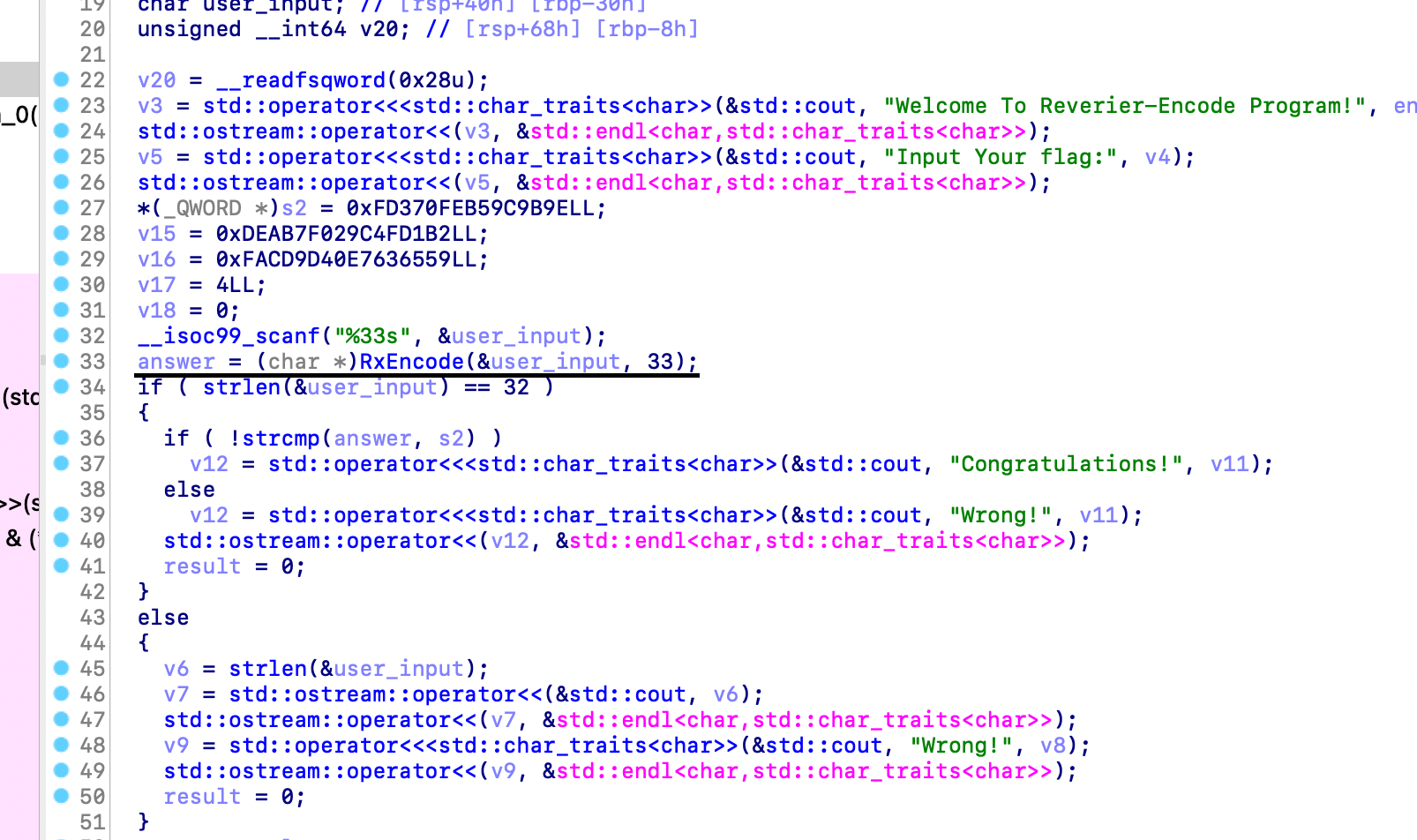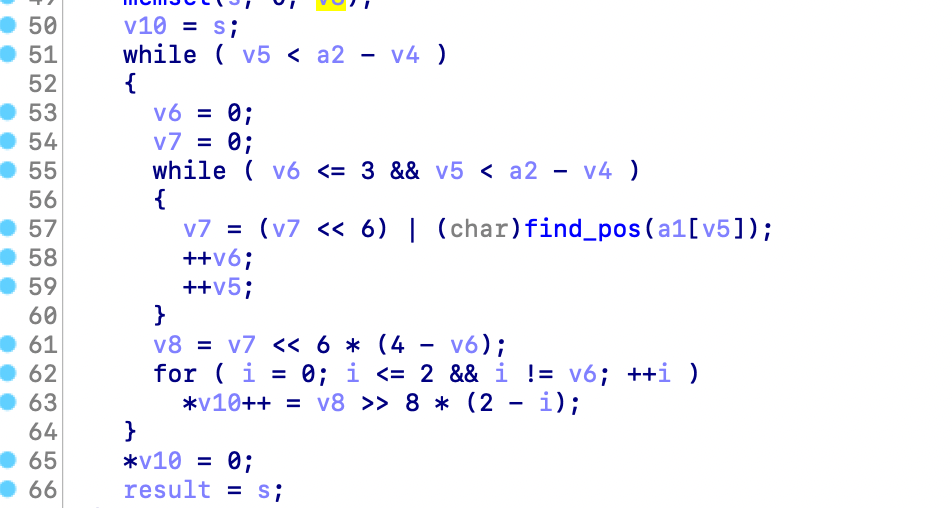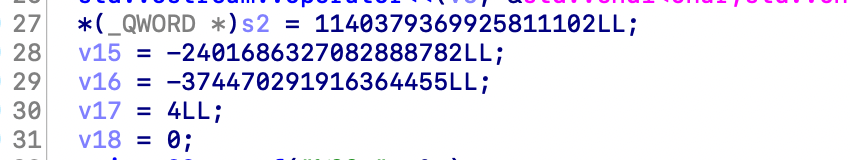# [NPUCTF2020]你好sao啊 题解

### 二进制文件信息获取### 静态分析if (user_input[lengthPlusOne - 1] == '=') v4 = 1;
if (user_input[lengthPlusOne - 2] == '=') ++v4;
if (user_input[lengthPlusOne - 3] == '=') ++v4;
if (v4 == 3) {
v3 += 2;
} else if (v4 <= 3) {
if (v4 == 2) {
v3 += 3;
} else if (v4 <= 2) {
if (v4) {
if (v4 == 1) v3 += 4;
} else {
v3 += 4;
}
}
}


v3 = 3 * (a2 / 4);


----------- 华丽的分割线 -------------------------------------------ABCDEFGHIJKLMNOPQRSTUVWXYZabcdefghijklmnopqrstuvwxyz01234{}789+/=

v5(数组下标) < a2(输入总长度) - v4(结尾 = 号的个数)### 上脚本干他

#include <iostream>
#include <cstring>

int main() {
unsigned long long s[] = {
0xFD370FEB59C9B9E,
0xDEAB7F029C4FD1B2,
0xFACD9D40E7636559,
0x4,
0x0
};

for (int i = 0; i < 25; i++) {
int c = (int) * ((unsigned char *) (s) + i);
std::cout << "\\x" << std::hex << c;
}
std::cout << std::endl;

return 0;
}


\x9e\x9b\x9c\xb5\xfe\x70\xd3\xf\xb2\xd1\x4f\x9c\x2\x7f\xab\xde\x59\x65\x63\xe7\x40\x9d\xcd\xfa\x4

# coding:utf-8
s = 'ABCDEFGHIJKLMNOPQRSTUVWXYZabcdefghijklmnopqrstuvwxyz01234{}789+/'
def My_base64_encode():
inputs = b'\x9e\x9b\x9c\xb5\xfe\x70\xd3\x0f\xb2\xd1\x4f\x9c\x02\x7f\xab\xde\x59\x65\x63\xe7\x40\x9d\xcd\xfa\x04'
# 将字符串转化为2进制
bin_str = []
for i in inputs:
x = str(bin(i)).replace('0b', '')
bin_str.append('{:0>8}'.format(x))
# print(bin_str)
# 输出的字符串
outputs = ""
# 不够三倍数，需补齐的次数
nums = 0
while bin_str:
# 每次取三个字符的二进制
temp_list = bin_str[:3]
if(len(temp_list) != 3):
nums = 3 - len(temp_list)
while len(temp_list) < 3:
temp_list += ['0' * 8]
temp_str = "".join(temp_list)
# print(temp_str)
# 将三个8字节的二进制转换为4个十进制
temp_str_list = []
for i in range(0, 4):
temp_str_list.append(int(temp_str[i*6:(i+1)*6], 2))
# print(temp_str_list)
if nums:
temp_str_list = temp_str_list[0:4 - nums]

for i in temp_str_list:
outputs += s[i]
bin_str = bin_str[3:]
outputs += nums * '='
print("Encrypted String:\n%s " % outputs)
My_base64_encode()


Encrypted String:
npuctf{w0w+y0U+cAn+r3lllY+dAnc3}BA==


posted @ 2021-06-06 18:32  Node_Sans  阅读(56)  评论(0编辑  收藏  举报